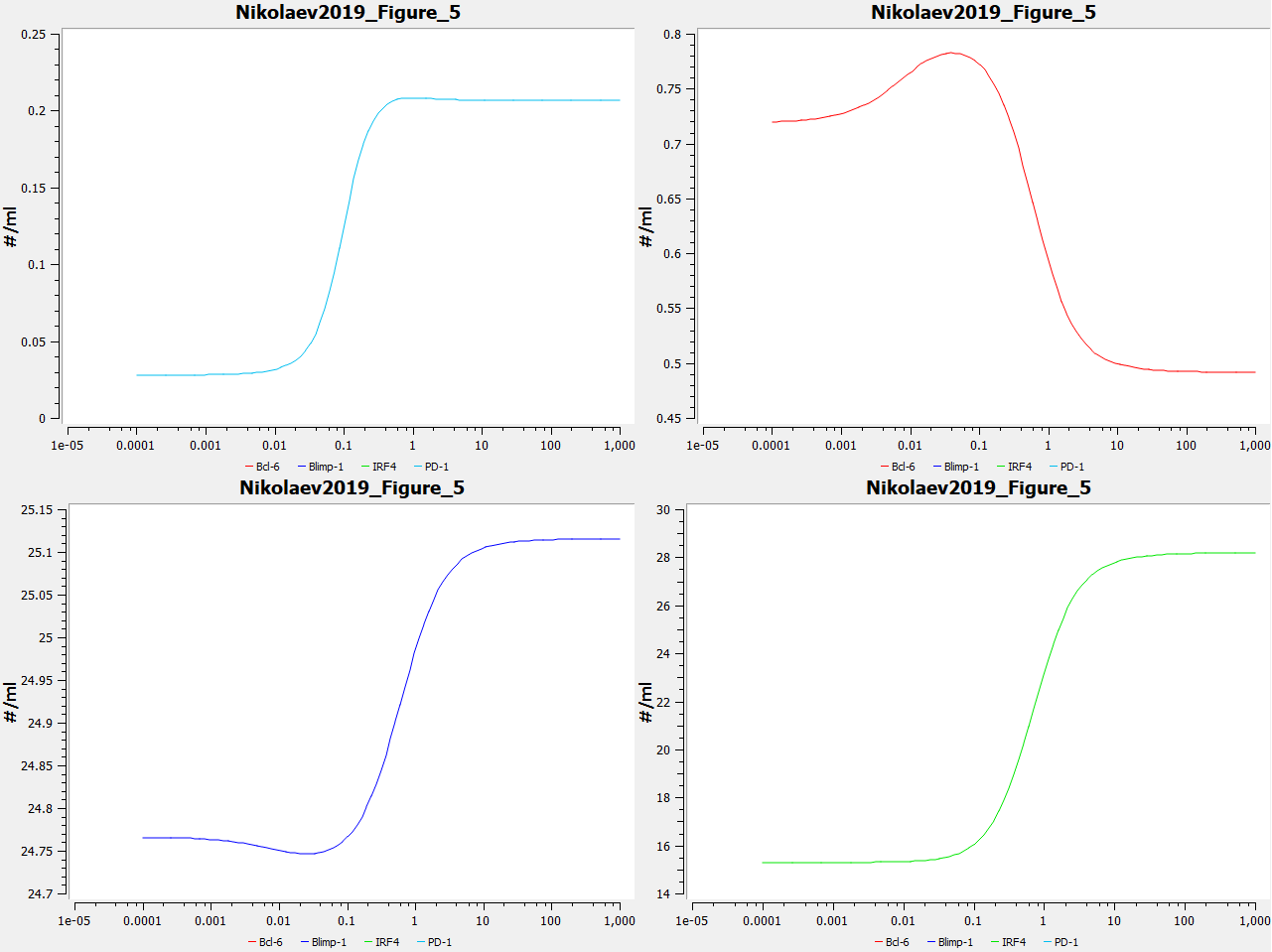## Nikolaev2019 - Immunobiochemical reconstruction of influenza lung infection-melanoma skin cancer interactionsModel Identifier
BIOMD0000000865
Short description
This is a mathematical mechanistic immunobiochemical model that incorporates T cell pathways that control programmed cell death protein 1 (PD-1) expression. A core component of the model is a kinetic motif, termed a PD-1 Double Incoherent Feed-Forward Loop (DIFFL), which reflects known interactions between IRF4, Blimp-1, and Bcl-6.
Format
SBML (L2V4)
Related Publication
• Immunobiochemical Reconstruction of Influenza Lung Infection-Melanoma Skin Cancer Interactions.• Nikolaev EV, Zloza A, Sontag ED
• Frontiers in immunology , 1/ 2019 , Volume 10 , pages: 4 , PubMed ID: 30745900
• Center for Quantitative Biology, Rutgers University, Piscataway, NJ, United States.
• It was recently reported that acute influenza infection of the lung promoted distal melanoma growth in the dermis of mice. Melanoma-specific CD8+ T cells were shunted to the lung in the presence of the infection, where they expressed high levels of inflammation-induced cell-activation blocker PD-1, and became incapable of migrating back to the tumor site. At the same time, co-infection virus-specific CD8+ T cells remained functional while the infection was cleared. It was also unexpectedly found that PD-1 blockade immunotherapy reversed this effect. Here, we proceed to ground the experimental observations in a mechanistic immunobiochemical model that incorporates T cell pathways that control PD-1 expression. A core component of our model is a kinetic motif, which we call a PD-1 Double Incoherent Feed-Forward Loop (DIFFL), and which reflects known interactions between IRF4, Blimp-1, and Bcl-6. The different activity levels of the PD-1 DIFFL components, as a function of the cognate antigen levels and the given inflammation context, manifest themselves in phenotypically distinct outcomes. Collectively, the model allowed us to put forward a few working hypotheses as follows: (i) the melanoma-specific CD8+ T cells re-circulating with the blood flow enter the lung where they express high levels of inflammation-induced cell-activation blocker PD-1 in the presence of infection; (ii) when PD-1 receptors interact with abundant PD-L1, constitutively expressed in the lung, T cells loose motility; (iii) at the same time, virus-specific cells adapt to strong stimulation by their cognate antigen by lowering the transiently-elevated expression of PD-1, remaining functional and mobile in the inflamed lung, while the infection is cleared. The role that T cell receptor (TCR) activation and feedback loops play in the underlying processes are also highlighted and discussed. We hope that the results reported in our study could potentially contribute to the advancement of immunological approaches to cancer treatment and, as well, to a better understanding of a broader complexity of fundamental interactions between pathogens and tumors.
Contributors
Submitter of the first revision: Johannes Meyer
Submitter of this revision: Johannes Meyer
Modellers: Johannes Meyer

hasTaxon (1 statement)
Taxonomy Mus musculus

hasProperty (2 statements)

Curation status
Curated

Modelling approach(es)

Tags

#### Connected external resources

SBGN view in Newt Editor

Name Description Size Actions

### Model files

Nikolaev2019.xml SBML L2V4 Representation of Nikolaev2019 - Immunobiochemical reconstruction of influenza lung infection-melanoma skin cancer interactions 63.80 KB Preview | Download

Nikolaev2019.cps COPASI file of Nikolaev2019 - Immunobiochemical reconstruction of influenza lung infection-melanoma skin cancer interactions 128.66 KB Preview | Download
Nikolaev2019.sedml SED-ML file of Nikolaev2019 - Immunobiochemical reconstruction of influenza lung infection-melanoma skin cancer interactions 9.51 KB Preview | Download
• Model originally submitted by : Johannes Meyer
• Submitted: Nov 18, 2019 9:19:02 AM
##### Revisions
Legends
: Variable used inside SBML models

Species
Species Initial Concentration/Amount
C

C26149
1.0 item
I

C17926
1.0 item
B

PR:000001831
1.0 item
P

PR:000001919
1.0 item
Reactions
Reactions Rate Parameters
C => compartment*mu_c*C mu_c = 0.1
=> I; B, I compartment*Phi_L_P*(sigma_i+a_i*U_a_k_P^n_i/(A_i^n_i+U_a_k_P^n_i)+k_i*B^m_i/(K_i^m_i+B^m_i)+q_i*I^s_i/(Q_i^s_i+I^s_i)) U_a_k_P = 0.0215814688039663; Q_i = 1.0; n_i = 2.0; a_i = 75.0; k_i = 7.5; m_i = 2.0; q_i = 7.5; s_i = 2.0; sigma_i = 0.3; K_i = 1.0; Phi_L_P = 1.0; A_i = 1.0
=> B; I, C compartment*(a_b*U_a_k_P^n_b/(A_b^n_b+U_a_k_P^n_b)+k_b*I^m_b/(K_b^m_b+I^m_b))*M_b^r_b/(M_b^r_b+C^r_b) U_a_k_P = 0.0215814688039663; n_b = 2.0; a_b = 100.0; A_b = 10.0; r_b = 2.0; m_b = 2.0; K_b = 1.0; M_b = 10.0; k_b = 25.0
=> P; B compartment*(sigma_p_tilde+a_p*U_a_k_P^n_p/(A_p^n_p+U_a_k_P^n_p))*M_p^r_p/(M_p^r_p+B^r_p) U_a_k_P = 0.0215814688039663; n_p = 3.0; sigma_p_tilde = 0.1; A_p = 0.1; a_p = 0.75; M_p = 10.0; r_p = 4.0
B => compartment*mu_b*B mu_b = 1.0
=> C; B, I compartment*a_c*U_a_k_P^n_c/(A_c^n_c+U_a_k_P^n_c)*M_c^r_c/(M_c^r_c+B^r_c+I^r_c+C^r_c) U_a_k_P = 0.0215814688039663; n_c = 3.0; A_c = 0.01; r_c = 2.0; M_c = 10.0; a_c = 0.75
I => compartment*mu_i*I mu_i = 1.0
P => compartment*mu_p*P mu_p = 0.1Curator's comment:
(added: 18 Nov 2019, 09:18:55, updated: 18 Nov 2019, 09:40:54)
Reproduced plots of Figure 5 in the original publication. Curves correspond to magenta-coloured curve in original publication (k_off = 1.0). Model simulated and plots produced using COPASI 4.24 (Build 197).